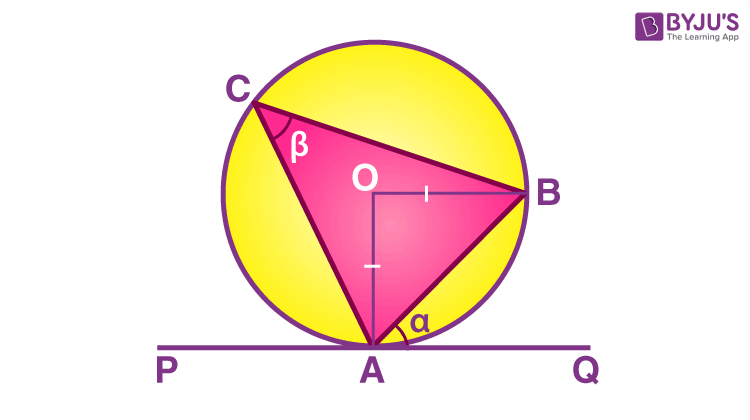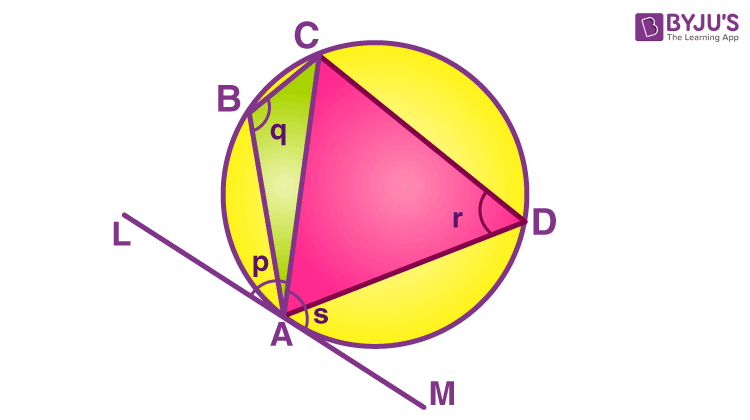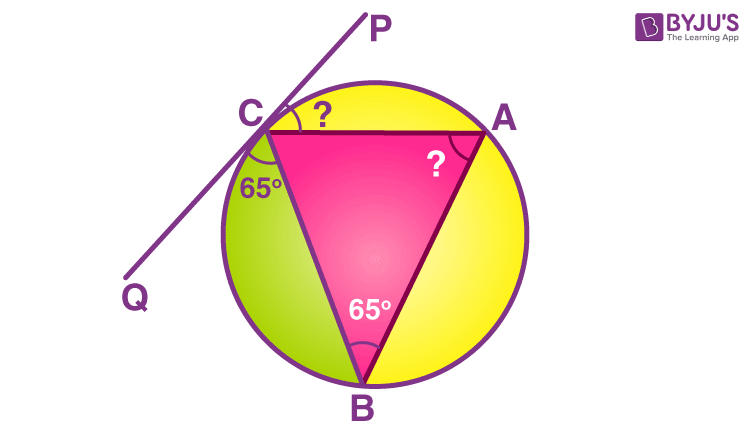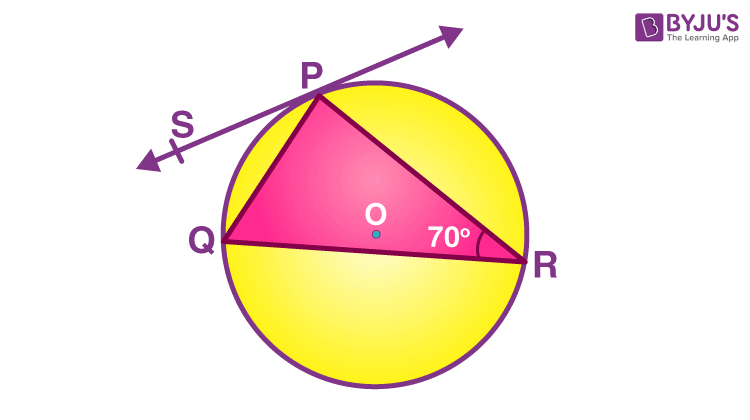# Alternate Segment Theorem

In Mathematics, we know that a circle is a two-dimensional figure, in which all the points on the surface of the circle are equidistant from the centre point. The circle’s segment is the region between the chord and the corresponding circle’s arc. There are different theorems related to the circle. In this article, we are going to discuss one of the theorems called “Alternate segment Theorem” with its statement, proofs, and solved examples.

## Alternate Segment Theorem Statement

The alternate segment theorem is one of the circle theorems. The theorem states that “For any circle, the angle formed between the tangent and the chord through the point of contact of the tangent is equal to the angle formed by the chord in the alternate segment”. The alternate segment theorem is also known as the tangent-chord theorem.

## Alternate Segment Theorem ProofLet us assume that the tangent is drawn to the circle, such that the point of contact is A.

Through A, a chord AB is drawn that should be inclined to the tangent at an angle “α”.

Suppose that AB subtends an angle β at point C anywhere on the surface of the circle as shown in the figure.

Assume that ∠ACB = ∠β is the alternate angle in the alternate segment for the angle between the tangent A and the chord AB.

Proof:

Let A be the point on the circumference of the circle, and “O” be the centre of the circle.

Assume that PQ is the tangent of the circle that passes through point A.

The tangent makes an angle α with the chord AB.

Now, consider that ∠ACB = ∠β in the alternate segment.

Now, we have to prove that ∠α =∠β.

Thus, OA =OB (Both are the radii of the circle)

Also, ∠OAB = ∠OBA (since the angles opposite to the equal sides are equal)

Since, OAB is an isosceles triangle

∠AOB = 180° – ∠OAB – ∠OBA

∠AOB = 180° – 2∠OAB …(1)

Since, the line segment PQ is the tangent line,

∠OAQ = 90°

Therefore, α= 90° – ∠OAB …(2)

From the equations (1) and (2), we can write

∠AOB = 2α

We know that the angle at the centre of the circle is twice the angle at the circumference of the circle.

∠AOB = 2∠ACB

∠ACB= (½)∠AOB

Now, substitute ∠AOB = 2α in the above equation, we get

∠β= (½) 2α

∠β= ∠α

Thus, the alternate segment theorem is proved.

The alternate segment theorem is applicable to find the alternate segments in the quadrilateral too.Consider the image given above,

By using the alternate segment theorem, we can say that

∠p = ∠r

Now, we need to prove ∠s = ∠q

As the tangent line, LM is straight, we get

∠p + ∠s = 180° …(3)

Since the angles ∠r and ∠q are the opposite angles in the cyclic quadrilateral, we can say

∠q + ∠r = 180° …(4)

Now, equate the equations (3) and (4), we get

∠p + ∠s = ∠q + ∠r

Thus, ∠p = ∠r and ∠s = ∠q.

Hence, proved.

### Solved Examples

Go through the following examples to understand the concept of the alternate segment theorem.

Example 1:

Find the unknown angles in the figure, given that the chord BC makes the angles 65° with the tangent line PQ.Solution:

Given that, ∠QCB = 65°

By using the alternate segment theorem, we can say

∠CAB = 65°

Similarly, by using the angles in the alternate segment, ∠PCA = 65°

Therefore,

∠CAB = 65° and ∠PCA = 65°.

Example 2:

Find the angle ∠QPS in the given figure.Solution:

Given that, ∠PRQ = 70°.

By using the alternate segment theorem, ∠R= ∠P.

(i.e.,) ∠QPS = ∠PRQ

Hence, ∠QPS = 70°.

## Frequently Asked Questions on Alternate Segment Theorem

### Is the alternate segment theorem a circle theorem?

Yes, the alternate segment theorem is one of the circle theorems.

### What is the other name of the alternate segment theorem?

The other name of the alternate segment theorem is called the tangent-chord theorem.

### Explain the alternate segment theorem.

The alternate segment theorem states that the measurement of the angle between the chord and the tangent through any of the endpoints of the chord is equal to the measurement of angle in the alternate segment.

### Mention a few circle theorems.

Some of the circle theorems are:
The angle at the centre theorem
Angle in a semicircle